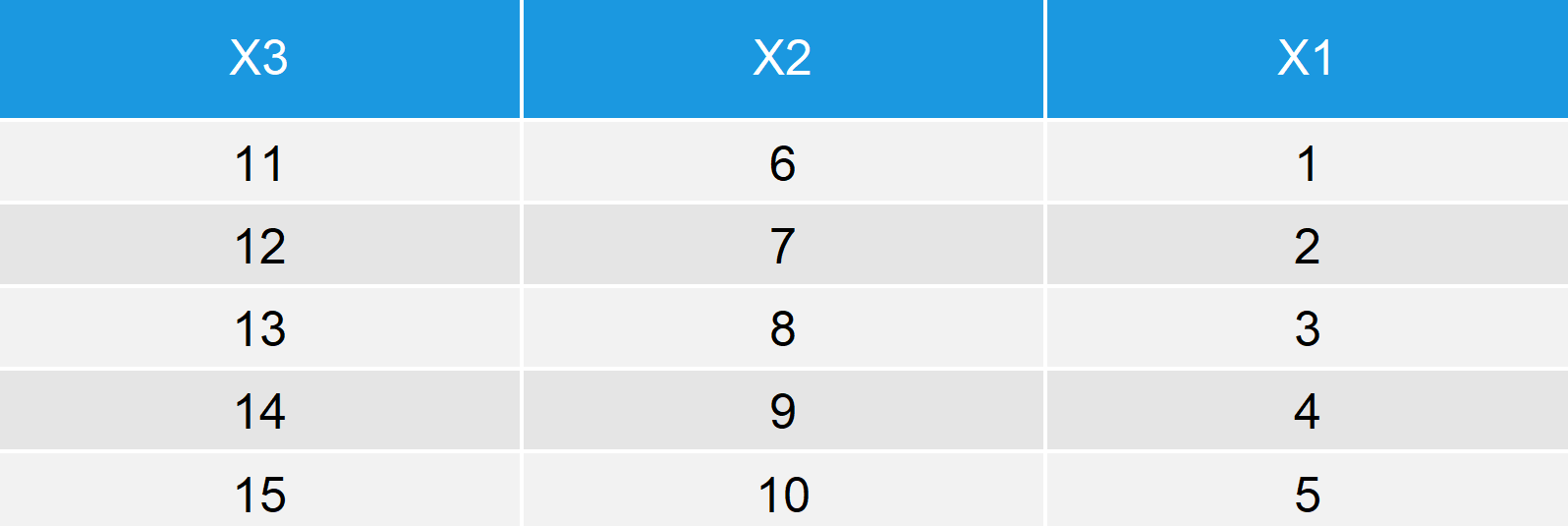# rev R Function | 3 Examples (Reverse of Vector, Data Frame by Column & by Row)

Basic R Syntax:

`rev(x)`

The rev R function returns a reversed version of a vector or other data objects. The basic R code for the rev command is illustrated above.

In this R tutorial, I’m going to show you three examples for the usage of rev in R.

Let’s start right away…

## Example 1: Reverse Vector via rev() R Function

Typically, the rev R function is used to reverse the order of vectors. Let’s create an example vector:

```vec <- 1:10                              # Create example vector
vec                                      # Print example vector to RStudio console
# 1  2  3  4  5  6  7  8  9 10```

Our example vector consists of ten numbers ordered from 1 to 10. Now, let’s reverse this vector with the rev() command:

```vec_rev <- rev(vec)                      # Apply rev function to vector
vec_rev                                  # Print reversed example vector
# 10  9  8  7  6  5  4  3  2  1```

As you can see, we reversed the order of our vector from 10 to 1.

Easy! But stay with me, there is more…

## Example 2: Apply rev to Data Frame to Reverse Columns

With the R rev function, we can also reverse the ordering of the columns of a data frame. Let’s create some example data:

```data <- data.frame(x1 = 1:5,             # Create example data.frame
x2 = 6:10,
x3 = 11:15)
data                                     # Print example data.frame to RStudio console```Table 1: Example Data Frame.

Our dataset has three columns containing the numbers 1 to 5, 6 to 10, and 11 to 15, respectively.

We can reverse the order of the columns with the following R code:

```data_rev <- rev(data)                    # Apply rev function to data.frame
data_rev                                 # Print reversed example data.frame```Table 2: Example Data Frame Reversed by Column.

As you can see, the positions of X1 and X3 are exchanged.

OK nice, so can we do that also by row? Yes, of cause we can:

## Example 3: Reverse Order of Rows

For the third example, I’m going to use the same data frame as in Example 2. We can reverse the order of the rows of this data frame with the following lines of code:

```data_rev_row_a <- apply(data, 2, rev)    # rev() & apply() functions combined
data_rev_row_a                           # Print data.frame _a reversed by row```

As you have seen, we used rev in combination with the apply function. You might say that’s too complicated – But no problem, there’s an even simpler solution which relies only on the nrow function:

```data_rev_row_b <- data[nrow(data):1, ]   # Alternative without rev()
data_rev_row_b                           # Print data.frame _b reversed by row```Table 3: Example Data Frame Reversed by Row.

## Video & Further Resources on the Topic

Do you need more explanations on how to apply the rev R function? Then you should have a look at the following YouTube video of the Statistics Globe YouTube channel, where I’m explaining the topics of this post:

Please accept YouTube cookies to play this video. By accepting you will be accessing content from YouTube, a service provided by an external third party.If you accept this notice, your choice will be saved and the page will refresh.

Still hungry? No worries, there is more to learn. In the following video tutorial of the LearnR YouTube channel you will see a bunch of further examples that you can use for your analytics in R programming – Not only to flip rows and columns with rev(), but also for related functions such as which(), order(), attach(), and matrix().

Please accept YouTube cookies to play this video. By accepting you will be accessing content from YouTube, a service provided by an external third party.If you accept this notice, your choice will be saved and the page will refresh.